## Example Questions

### Example Question #1 : How To Find A Ratio Of Exponents

Quantitative Comparison

Quantity A: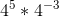Quantity B: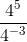Quantity A is greater.

The two quantities are equal.

Quantity B is greater.

The relationship cannot be determined from the information given.

Quantity B is greater.

Explanation:

In Quantity A, we add the exponents because the operation is multiplication, so 45 * 4–3 = 45+(–3) = 42. In Quantity B, we subtract the exponents because the operation is division, so 45 / 4= 45–(–3) = 48. We don't have to finish multiplying out the exponents to see that Quantity B is greater.

### Example Question #1 : How To Find A Ratio Of Exponents

If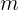andare both rational numbers and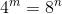, what is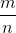?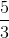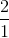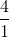Explanation:

This question is asking you for the ratio of m to n.  To figure it out, the easiest way is to figure out when 4 to an exponent equals 8 to an exponent.  The easiest way to do that is to list the first few results of 4 to an exponent and 8 to an exponent and check to see if any match up, before resorting to more drastic means of finding a formula.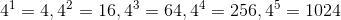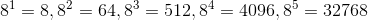And, would you look at that.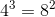.  Therefore,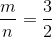.

### All GRE Math Resources